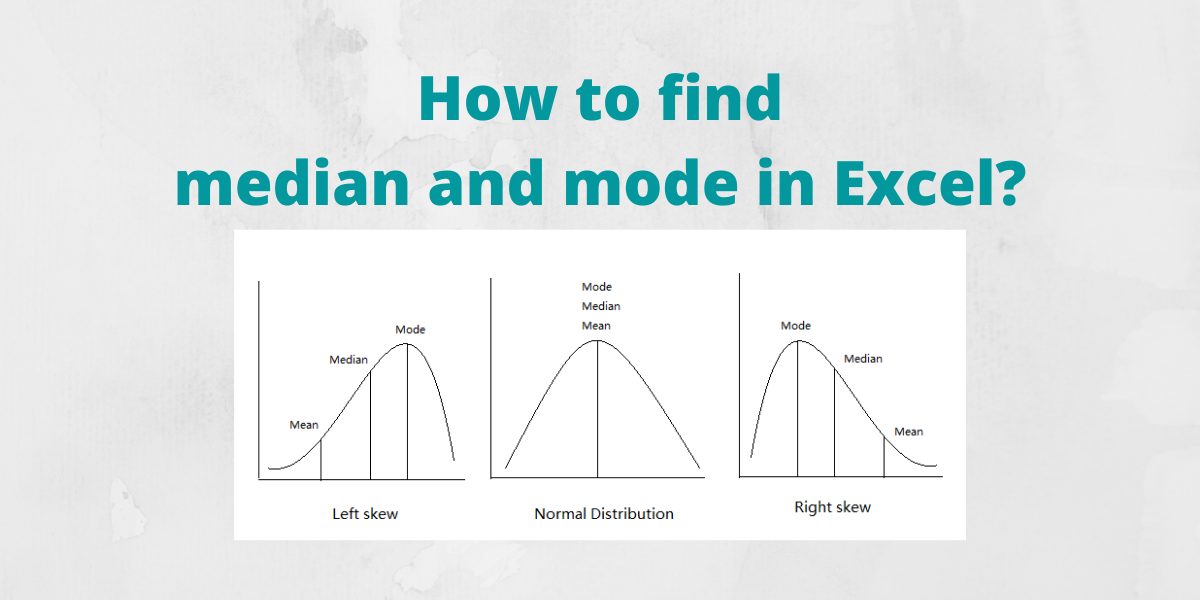# How to Find Median and Mode in Excel?Today, we’ll learn to find the median and mode in Excel. In statistics, the median is the value that separates the upper and lower halves of a data sample or population or simply the middle number in a sorted list of numbers. Excel has an inbuilt function MEDIAN to find the median value of a dataset.

Also read: How to calculate mean in Excel? [Easy Step-by-Step Method]

## Using the MEDIAN function

The MEDIAN function returns the median of a list of numbers. Syntax: =MEDIAN(n), where n is the list of numbers or reference to the cell ranges containing the numbers.

Let us consider a list of numbers whose median we are interested in calculating:

• Go to the cell where you want to display the median value.
• Type =MEDIAN(, select the cells containing the numbers and complete the formula with ).
• Press Enter key to display the calculated median value.

Note:

• The Excel MEDIAN function returns the middle number in the dataset when the total number of items is odd. It returns the average of the two middle numbers when the total number of items in the dataset is even.
• In calculating median using the MEDIAN function, cells with values as zero (0) are included.
• The MEDIAN function ignores empty cells as well as cells containing text or logical values.
• TRUE and FALSE logical values put directly into the MEDIAN function’s parameters are counted as 1 and 0 respectively. The formula MEDIAN(FALSE, TRUE,2,3,4), returns 2 as the dataset is read as {0,1,2,3,4}.

### Using the MEDIAN IF formula

Sometimes you want to exclude zero values while calculating the median of a dataset. Excel does not have a dedicated function to do this, but a workaround is available using a MEDIAN IF formula. The syntax for this formula is MEDIAN(IF(criteria_range=criteria, cell_ranges)), where criteria are the condition to be satisfied by the values in the cells, cell_ranges is the reference to the cell ranges containing the values.

Let us consider a list of numbers whose median we are interested in calculating excluding zero values:

• Go to the cell where you want to display the median value.
• Type =MEDIAN(IF(A1:A10>0,A1:A10)).
• Press Enter key to display the calculated median value of the numbers excluding zero values.

To illustrate the difference in the median, we calculated the mean of the above dataset including zeroes.

As you can see, the median is different in both cases.

## Finding mode in Excel

The mode is a statistical term that refers to the most frequently occurring number found in a set of numbers. A data set can have none, one, or multiple modes. In Excel, there are three functions to find the mode/modes of a dataset which are discussed below:

### Using the MODE function

This function returns a single mode. Syntax: MODE(n), where n is the list of numbers or reference to the cell ranges containing the numbers. Follow these steps to find the mode of a dataset using the MODE function:

• Go to the cell where you want to display the mode value.
• Type =MODE(, select the cells containing the numbers and complete the formula with ).
• Press Enter key to display the result.

Note:

• If the data set contains no data value that has at least one duplicate value, the MODE function returns the #N/A error value.
• The MODE function ignores empty cells as well as cells containing text or logical values.

### Using the MODE.MULT function

This function returns more than one result if there are multiple modes in the dataset. Syntax: MODE.MULT(n), where n is the list of numbers or reference to the cell ranges containing the numbers. Follow these steps to find the Mode of a dataset using the MODE.MULT function:

• Go to the cell where you want to display the mode value.
• Type =MODE.MULT(, select the cells containing the numbers and complete the formula with ).
• Press Enter key to display the result.

Note:

• If the data set contains no data value that has at least one duplicate value, the MODE.MULT function returns the #N/A error value.
• The MODE.MULT function ignores empty cells as well as cells containing text or logical values.

### Using the MODE.SNGL function

This function is an advanced version of the MODE function and returns a single most frequently occurring value in a dataset. Syntax: MODE.SNGL(n), where n is the list of numbers or reference to the cell ranges containing the numbers. Follow these steps to find the mode of a dataset using the MODE.SNGL function:

• Go to the cell where you want to display the mode value.
• Type =MODE.SNGL(, select the cells containing the numbers and complete the formula with ).
• Press the Enter key to display the result.

Note:

• If the data set contains no data value that has at least one duplicate value, the MODE.SNGL function returns the #N/A error value.
• The MODE.SNGL function ignores empty cells as well as cells containing text or logical values.

## Conclusion

We discussed simple and quick ways of calculating the median and the mode of a dataset in Excel.